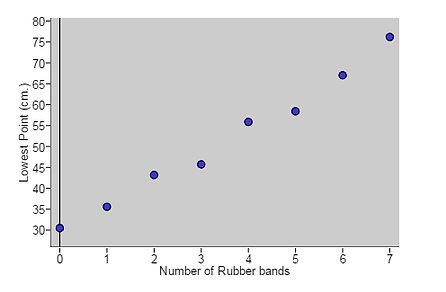## Day 23 - Lesson 2.4

##### Learning Targets
• Calculate the correlation between two quantitative variables.

• Apply the properties of the correlation.

• Describe how outliers influence the correlation.

##### Activity:Today the students will be discovering properties of correlation.  We are not requiring students to calculate correlation so they will be using the applet for that. We began by giving the students a set of Barbie Bungee data.  In order to give the r value relevance and meaning, we used it to discuss the students’ level of confidence on how sure they were that Barbie wouldn’t die on her jump.

The group data we gave students was measured in inches when it should have instead been in centimeters. This gave us a context for looking at how the correlation does not change when you switch the units (multiply all the values by a constant).  When we asked students to explain why they thought the correlation stayed the same, they said, “The line didn’t change, it just moved up.  The points are still the same distance apart.”  Thinking about the change in the data as a shift makes the property easier to explain.  We didn’t have an example in the activity of how the correlation does not change when you switch the axes so you will want to draw attention to this in the text.

Lastly, students examined the effects of outliers on the correlation.  Students found that outliers within the linear pattern actually made the correlation stronger while the outliers outside of the pattern weakened it.##### Notes

Having the students find the properties of correlation was helpful.  We always prefer to have students discover on their own as opposed to us telling them the information.  They will have a deeper understanding of the content which will help them to remember it longer as well.